Courses
Courses for Kids
Free study material
Free LIVE classes
More

# Average and RMS Value for JEELIVE
Join Vedantu’s FREE Mastercalss

## An Introduction to Average and RMS Value

Average Value and RMS value are important expressions for sinusoidal waves. As we know that there are two types of sources and currents: the AC source and DC source; AC and DC. The AC or Alternating Currents is the one that repeats itself after 2π, whereas the DC current source is the one that does not alternate. Some important terms associated with both AC and DC are: RMS value, instantaneous value and the average value.

RMS value is the amount of heat produced by a resistor when at a time both AC and DC are passed through it. Average value is the area of the one cycle of sinusoidal wave to the time period. However, instantaneous value is the value at a particular instant.  Let us further understand these concepts below.

## RMS Value of Current

RMS or the root mean square value of current is the amount of heat produced by a resistor or circuit when an AC and DC pass through it. If we assume the RMS value of currents as Irms and its peak value is Im, then the RMS value formula is given as:

$I_{r m s}=\dfrac{I_{m}}{\sqrt{2}}$

This is also referred to as the RMS value of alternating current, where Im is the maximum value of the sine wave.

## RMS Value of Sine Wave

We have already seen the expressions for the RMS values but we will now derive the RMS value using the analytical method. Let’s first derive the RMS value of current and then we can generalise it for voltage too. Since the sine wave is symmetrical, we can calculate the RMS value by considering the half cycle only.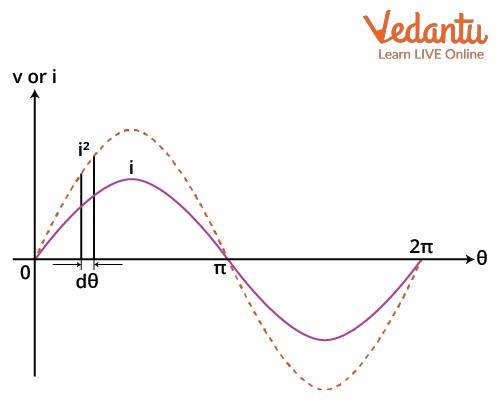Sinusoidal Wave

We know that the equation of a sinusoidal alternating current i is given as

$i=I_{m} \sin \theta$.

Since the current changes from positive to negative in a n AC signal, we will use the squared current wave because it is always positive for our calculations.

Let’s consider i2 to be the average height of the strip in the above figure. The area of this strip A will be

$A=i^{2} d \theta$

The area of the half cycle of i2 wave will be given as

\begin{align} &\int A_{h}=\int_{0}^{\pi} i^{2} \mathrm{~d} \theta \\ &A_{h}=\int_{0}^{\pi} I_{m}^{2} \sin ^{2} \theta \mathrm{d} \theta \\ &A_{h}=\dfrac{I_{m}^{2}}{2} \int_{0}^{\pi}(\cos 2 \theta+1) \mathrm{d} \theta \\ &A_{h}=\dfrac{I_{m}^{2}}{2}\left[\theta-\dfrac{\sin 2 \theta}{2}\right]_{0}^{\pi} \\ &A_{h}=\dfrac{\pi I_{m}^{2}}{2} \end{align}

The mean i.2 or the mean square current can be found by dividing the area by the period or in this case $\pi$. So the mean square current will be

\begin{align} \overline{i^{2}} &=\dfrac{\dfrac{\pi I_{m}^{2}}{2}}{\pi} \\ \overline{i^{2}} &=\dfrac{I_{m}^{2}}{2} \end{align}

The root mean square current will be given by the root of the mean square current. This will be

\begin{align} &I_{R M S}=\sqrt{\dfrac{I_{m}{ }^{2}}{2}} \\ &I_{R M S}=\dfrac{I_{m}}{\sqrt{2}} \end{align}

This is the expression for the root mean square current. This whole derivation can be repeated for the voltage too and the root mean square voltage will also be

$V_{R M S}=\dfrac{V_{m}}{\sqrt{2}}$

As discussed above the RMS value of a sine wave is denoted as $I_{r m s}=\dfrac{I_{m}}{\sqrt{2}}$ or for sinusoidal voltage it is $V_{r m s}=\dfrac{V_{m}}{\sqrt{2}}$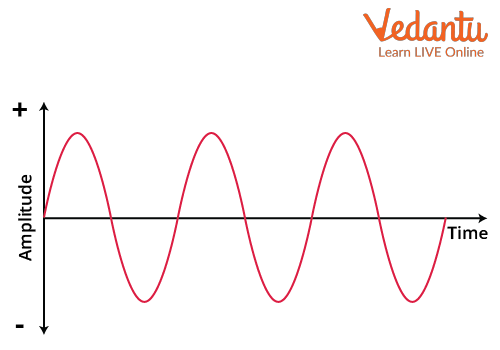Alternating Current or AC

Im and Vm here are the peak value of current and voltage respectively. Vm or Im  from above can be calculated as Vrms $\cdot \sqrt{2}$

## RMS Value of Square Wave

As we have calculated the RMS value of sinusoidal waves, similarly, we will calculate the RMS value of a square wave. The RMS value is basically just the root of the mean square value. Suppose we have a square wave as given below.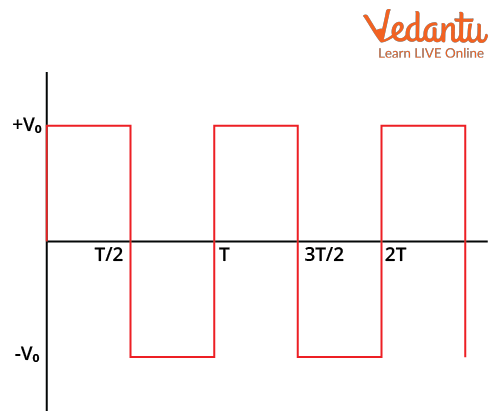A square wave

The mean square of this wave form can be given as

$\overline{V^{2}}=\dfrac{\int_{0}^{T} V^{2} \mathrm{~d} t}{T}$

We can solve this integral by breaking it into two parts from 0 to $\dfrac{T}{2}$ and then from $\dfrac{T}{2}$ to T. This will give

\begin{align} &\overline{V^{2}}=\dfrac{1}{T}\left(\int_{0}^{\dfrac{T}{2}} V_{0}^{2} \mathrm{~d} t+\int_{0}^{\dfrac{T}{2}}\left(-V_{0}\right)^{2} \mathrm{~d} t\right) \\ &\overline{V^{2}}=\dfrac{1}{T}\left(V_{0}^{2}\right)\left([t]_{0}^{T / 2}+[t]_{T / 2}^{T}\right) \\ &\overline{V^{2}}=\dfrac{V_{0}^{2}}{T}\left(\left[\dfrac{T}{2}-0\right]+\left[T-\dfrac{T}{2}\right]\right) \\ &\overline{V^{2}}=\dfrac{V_{0}^{2}}{T^{2}} \cdot T \\ &\overline{V^{2}}=V_{0}^{2} \end{align}

This is the mean square, now the root mean square voltage will be given as

\begin{align} &V_{R M S}=\sqrt{V_{0}^{2}} \\ &V_{R M S}=V_{0} \end{align}

This means that the rms value of a square wave is the same as its magnitude. Suppose the magnitude of a square wave is A, then its rms value will also be equal to A.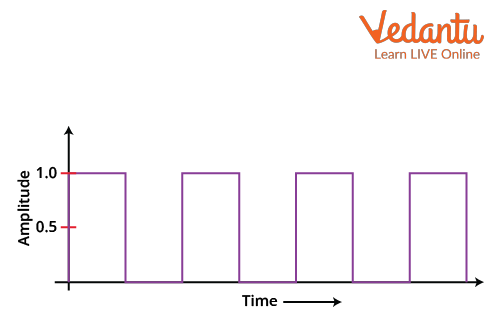A square wave

## Average Value of AC

When AC is converted to DC using a rectifier, this converted value of AC is known as the average value of AC. The average value of a sinusoidal wave can be calculated graphically or using the standard sinusoidal equation. By using the standard sinusoidal equation, the average value of AC comes out to be $I_{\text {average }}=\dfrac{2 I_{m}}{\pi}$, where Im is the peak value of the sinusoidal wave.

## Average Value of AC Formula

We will now find the average value for any AC signal. Let’s consider the graph of an AC sinusoidal waveform.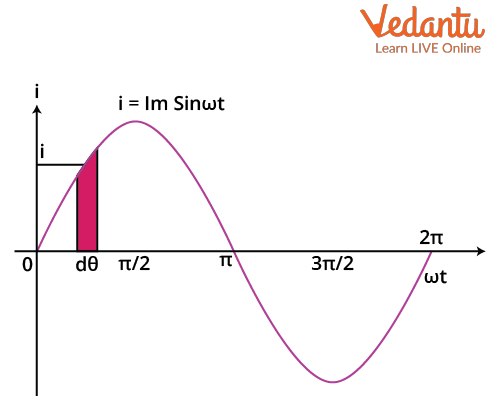AC Sinusoidal Waveform

Now the average can be found by dividing the area under the graph of the sine wave by the total time period for which the area has been found.

The average for the full cycle will be given as

\begin{align} &\bar{i}=\int_{0}^{T} i \mathrm{~d} t \\&\bar{i}=\int_{0}^{T} I_{m} \sin \omega t \mathrm{~d} t \\ &\bar{i}=I_{m}\left[\frac{-\cos \omega t}{\omega}\right]_{0}^{T} \\ &\bar{i}=\dfrac{I_{m}}{\omega}[-\cos \omega T-(-\cos 0)]\end{align}

Now we know that the time period is related to the angular frequency as

$T=\dfrac{2\pi}{\omega}$

Substituting this in the above expression gives,

\begin{align} &\bar{i}=\dfrac{I_{m}}{\omega}[-\cos 2 \pi+1] \\ &\bar{i}=0 \end{align}

So the average value of AC for a full cycle is zero.

For half cycle similarly we can find the average as

\begin{align} &\bar{i}=\int_{0}^{\dfrac{T}{2}} i \mathrm{~d} t \\&\bar{i}=\int_{0}^{\dfrac{T}{2}} I_{m} \sin \omega t \mathrm{~d} t \\&\bar{i}=I_{m}\left[\dfrac{-\cos \omega t}{\omega}\right]_{0}^{\dfrac{T}{2}} \end{align}

\begin{align} &\bar{i}=\dfrac{I_{m}}{\omega}\left[-\cos \left(\omega \dfrac{T}{2}\right)-(-\cos 0)\right]\\ &\bar{i}=\dfrac{I_{m}}{\omega}\left[-\cos \left(\omega \dfrac{2 \pi}{2 \omega}\right)+1\right] \\&\bar{i}=\dfrac{I_{m}}{\omega}[-\cos (\pi)+1] \\&\bar{i}=\dfrac{I_{m}}{\omega}[-(-1)+1] \\ &\bar{i}=\dfrac{2 I}{\omega} \end{align}

The average value of the AC formula is thus given as $I_{\text {average }}=\dfrac{2 I_{m}}{\pi}$. We calculated that the average value for a full sine wave is zero. As there is the same amount of current in the positive and negative cycle, and flowing in opposite directions thus, it neutralises the effect and the average value of a full sinusoidal wave is zero. The same formula holds true for an alternating voltage. Thus, the average value of the voltage formula is given as $V_{\text {average }}=\dfrac{2 V_{m}}{\pi}$.

W

It is true for a half cycle, for a full cycle, however, the average value of voltage is zero.

## Instantaneous Value

The instantaneous value of AC, on the other hand, is its value at a particular instant. In one cycle, there are multiple instances of a wave. Look at the figure below.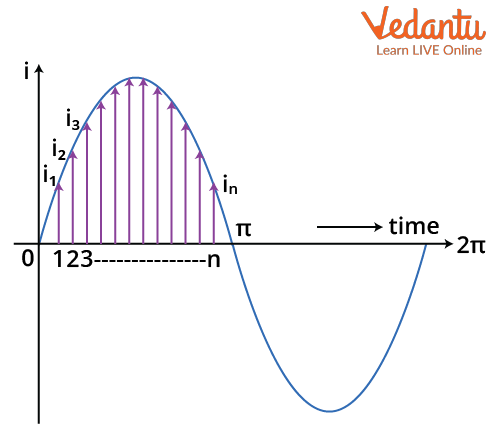Instantaneous Value of a Sine Wave

Now, we know that the current in a sine wave can be expressed in terms of its amplitude Im as

$i=I_{m} \sin \omega t$

For any value of t we can find the value of the current. This means that this is the formula for the instantaneous current at a particular value of t. If we want to find the value of current for a particular angle $\theta=\omega t$, then we can use the relation between the time period and angular frequency which is given as

$T=\dfrac{2\pi}{\omega}$.

This way we can find the instantaneous value of AC.

## Conclusion

AC and DC are fundamental supply sources in an electric circuit, be it the respective voltage or the currents. AC as we know is important as the supply to our homes is AC, as well as the appliances. However, it does not neglect the DC supply as it too is used for various applications. Electronic circuits such as TV Clock, laptop, LED strip etc all use DC supply. Moreover, solar panels themselves produce DC. With different sources comes the ways to denote them. Thus average , rms and instantaneous values play an important role. Each one of these quantities have their own significance which are as discussed above.

Last updated date: 15th Sep 2023
Total views: 127.8k
Views today: 2.27k

## FAQs on Average and RMS Value for JEE

1. Is the supply of AC voltage coming to our households of the rms value or the peak value?

The value of supply being fed to our is 440 volts peak or 230 volts RMS. This value of supply voltage operates or is denoted at a frequency of 50 Hz. The frequency varies from one country to another. In India, the frequency for supply is 50 hz with a tolerance limit of +0.5 or -0.5. Usually, the supply coming to our homes is denoted as the RMS value. For a household with a single phase supply, the value of supply voltage is 230 volts. Its peak value can be calculated from the relation given above.

2. What is the significance of RMS value and where is it used in day-to-day applications?

RMS value in an electric circuit produces the same amount of heat as an AC when passed through a resistor. Thus, from this, we can understand that the RMS value of an AC wave is equal to the DC source of the same value as AC producing the same heat.

One of the major applications of a RMS value of supply is that it is used in households. In India, the RMS valueof supply voltage is 220 volts, where in the USA it is 110 volts. The difference in the two is due to the standard frequency used in both the countries. Wecan also use RMS value in an electric circuit to find the equivalent DC value.